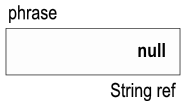taylorialcom/ Fundamentals

# Primitives versus References

Understanding the difference between primitives and reference types is critical.

When writing programs, it is often necessary to have variables that allow us to store values. A variable is a label that can be used in our program to refer to a particular value. This value can change as the program is executed. For example, the following Java code declares a variable with the label number, gives it the value 5, adds 3 to it, and then displays the new value of the variable on the console:

int number = 5;
number = number + 3;
System.out.println(number);


Java has two categories of variables: primitives and reference types.

## Primitives

A primitive is type that is built into Java. There are a number of different primitives. Some popular ones are shown in the table below.

Primitive TypeDescription
intRepresents an integer value — uses 4 bytes to store an integer value between $$-2,147,483,648$$ and $$2,147,483,647$$
doubleRepresents a decimal value — uses 8 bytes to store a decimal value (may have digits to the right of the decimal) between $$-1.79769313486231570 \times 10^{308}$$ and $$1.79769313486231570 \times 10^{308}$$ that could be as small as $$4.94065645841246544 \times 10^{-324}$$
charRepresents an individual character value — uses 2 bytes to store a Unicode value
booleanRepresents a boolean value — either true or false

Whenever a primitive is declared in Java, the type of value that the variable will represent must be specified. Therefore, declaring a primitive takes this form:

[TYPE] [VARIABLE_NAME];


where [VARIABLE_NAME] is the name of the variable and [TYPE] is the type of value the variable will contain. Consider:

int number;
number = 5;


The first line creates an integer variable called number,1 and the second line assigns the value 5 to the variable. We represent this graphically like this: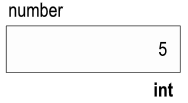## Reference Types

Java is an object oriented programming language. An object represents a more complicated chunk of data than what is stored in a primitive.2 We can create objects in Java and the variables that refer to objects are called reference variables. A reference variable stores an address. The address is the location of the object to which the reference variable refers.

There are many different types of objects. The type on a reference variable specifies the type of object the variable is intended to refer to. Whenever a reference is declared in Java, the type of object that the variable will refer to must be specified. Therefore, declaring a reference takes this form:

[TYPE] [VARIABLE_NAME];


where [VARIABLE_NAME] is the name of the variable and [TYPE] is the type of object the variable will refer to. Consider:

String phrase;
phrase = "that makes sense";


The first line creates a reference variable called phrase3, and the second line assigns the memory address of the String object containing that makes sense to the reference variable. We represent this graphically like this: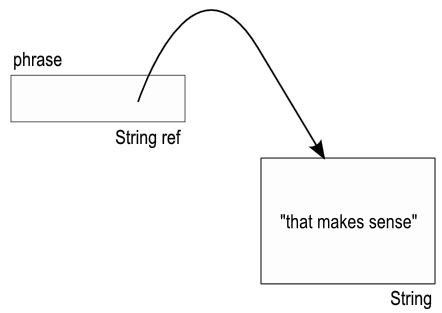## Who Cares?

Okay, so why am I making such a big deal about what seems like a pretty small difference here? Because it is not a small difference. Consider:

int number = 5;
int number2 = number;
String phrase = "that makes sense";
String phrase2 = phrase;


Here number2 gets a copy of the value stored in number. Likewise, phrase2 gets a copy of the value stored in phrase. The difference is that I've made a copy of the primitive stored in number, but I made a copy of the address stored in phrase — not the actual object. Graphically we represent the above code like this: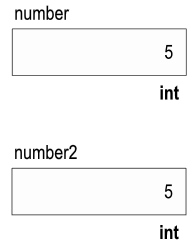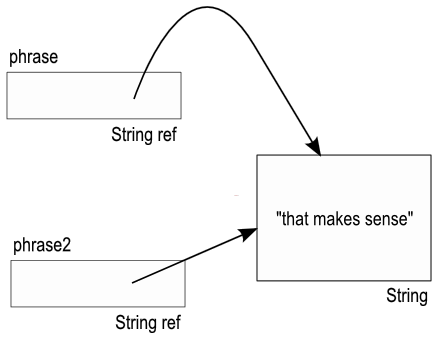1

Said more precisely, it claims four bytes of computer memory to hold a value that is interpreted using the rules associated the int primitive type and associates the label number with that location in memory.

2

This is a gross simplification. An object is more than just data. The object may also have behaviors, i.e., functionality, defined that it can perform on the data that it represents, but this is a useful simplification for now.

3

After declaring phrase in line 1 but before assigning a memory address to it in line 2 phrase contains a special value, null which indicates that it is not referring to any object. We represent this graphically like this: